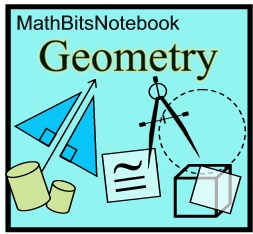Geometry section of MathBitsNotebook.comCirclesTangents and Circles (definition, common tangents, theorems)
Rules for Chords, Secants, Tangents (formulas for segments)
Angles and Circles (central, inscribed, tangent chord, 2 chords, 2 secants, 2 tangents, secant-tangent)
For constructions involving circles see Constructions.

 PRACTICE: • Practice with Chords • Practice with Tangents • Practice with Segments and Circles: Chords, Secants, Tangents • Practice with Central and Inscribed Angles • Practice with Tangent/Chord and Intersecting Chords • Practice with Angles Outside the Circle • Practice with BIG Circles • Practice with Arc Length and Radian Measure • Practice with Area of Sectors and Segments • Practice with Circle Proofs

 REFRESHERS: (while these topics are mostly "review", you may also find new information here) • Basic Circle Information (parts of circles, )

 EXTRAS: (interesting information - not necessarily appearing on assessments) • Power of a Point Theorem (under construction)